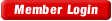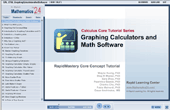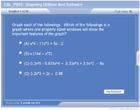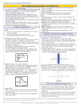HOME COURSES PREVIEW REVIEW ABOUT CONTACTTOLL-FREE INFO & ORDERING: M-F: 9am-5pm (PST): (877) RAPID-10

 24/7 Technical Support:

 Quick Search: Keywords:

 Rapid Learning Member Area:Note: If you are a legacy user of chemistry24 members, please request a new login access to the premium server with your full name and old login email via vip@rapidlearningcenter.com

 Rapid Courses Catalog : Physics in 24 Hours Chemistry in 24 Hours Biology in 24 Hours Mathematics in 24 Hours

Weekly Physics Tips:
Want to become a top gun in your class? How about study less yet score high? Sign up this Physics Survival Weekly to learn how. Designed specifically for students who are taking physics, this free newsletter will show you how to survive and excel in class! Weekly topics include:
• How to Study Physics Effectively
• How to Read Physics Textbooks Easily
• How to Solve Physics Problems Systematically
• How to Score High on Physics Exams Strategically
• How to Master Physics Rapidly
Each week, you will receive study tips on the topics above and visual tutorial or study template to enhance your physics learning. Enter your name and email below to subscribe free:

 Your Name * Email *

 Physics Study Lounge These study sheets are for quick review on the subjects. Refer to our rapid courses for comprehensive review.     - Basic Skills in Physics     - Basic Math for Physics     - How to Solve Physics Problems     - Newton's Laws Study Guide     - ElectroMagnetism Quick Review     - Atomic Physics At-A-Glance     - Core Concepts in Nuclear Physics     - Special Relativity Overview

 Other Related Sites
 Note: For course links to launch, disable popup blockers or hold the ctrl key while clicking the link.Home » College Calculus

Graphing Utilities and Math Software

 Topic Review on "Title": Graphing Aids: A graphing calculator is any handheld device that allows the user to input equations in “y=” form and will output an image of the graph for that input. CASs: Software run on a computer that allows the user to input equations and will provide a graphical image for that input.  Fixed Window: Plotting graphs using a pre-selected range of x and y values. Automatic Window: Plotting graphs using a pre-selected range of x values where the ranges of y values are calculated such that al points fit in the window.

Rapid Study Kit for "Title":
 Flash Movie Flash Game Flash Card Core Concept Tutorial Problem Solving Drill Review Cheat Sheet"Title" Tutorial Summary : Sometimes in a Calculus course it is helpful to use graphing utilities to graph difficult functions such as the ones with repeating values. Graphing calculators are needed to approximate solutions to some Calculus problems such as Taylor approximation. Using graphing calculators and mathematical software such as MAPLE, Mathematica and FORTRAN are used in a typical Calculus course to handle long calculations and graphs of difficult areas and volumes. The use of mathematical software creates a degree of error on some calculation. The bound of an error in approximating Taylor polynomials will be discussed in more detail later on.

 Tutorial Features: Specific Tutorial Features: The plots of equations are generated using a series of steps and guidelines. Examples showing the different characteristics of some particular plots of equations are covered in this tutorial with the help of examples. Series Features: Concept map showing inter-connections of new concepts in this tutorial and those previously introduced. Definition slides introduce terms as they are needed. Visual representation of concepts Animated examples—worked out step by step A concise summary is given at the conclusion of the tutorial.

 "Title" Topic List: ```Graphing Calculators Mathematical Software programs used in a Calculus course How to use graph functions using a graphing calculator and mathematical software? Graphing special functions and their unique characteristics ```

See all 24 lessons in college calculus, including concept tutorials, problem drills and cheat sheets:
Teach Yourself College Calculus Visually in 24 Hours